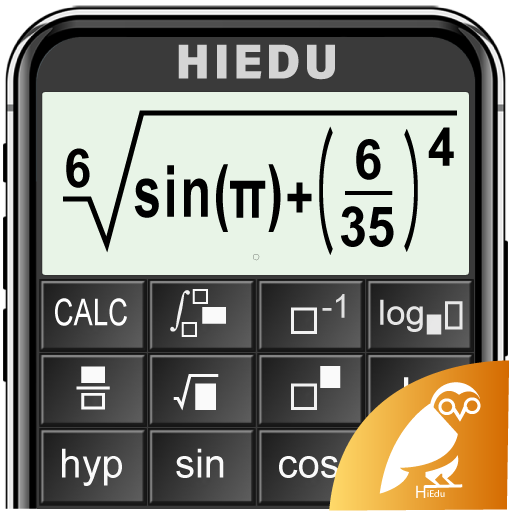# HiEdu Scientific Calculator

4.3.8 for Android
4.7 | 1,000,000+ Installs | Reviews

HiEdu - Development scientific calculator

⚠️ You need 9Apps App to install .XAPK File. How to install XAPK?

## Description of HiEdu Scientific Calculator

HiEdu Scientific Calculator He-570: solver app provides help with a variety of problems including arithmetic, algebra, trigonometry, calculus, statistics, complex, matrix, vector and other topics using an advanced AI powered math solver. Simply input a problem on app. HiEdu Scientific Calculator problem solver instantly recognizes the problem and helps you to solve it with 🔥FREE Step-By-Step Explanations🔥. Quickly look up related concepts. Get help with your homework problems and gain confidence in mastering 💯 the techniques 🔥It is absolutely FREE🔥
KEY FEATURES
Scientific calculator
• It is a scientific calculator app supports most of the features of real calculators: calculator for fractions, calculator algebra, calculator derivative, calculate integral, calculate differential, calculator square root, calculator with exponents, calculator with log, calculator with remainder, calculator division, calculator with variables,.. In addition, the calculator has factorial, absolute, combination, permutation... help you calculate easily
• Natural Display makes it possible to input and display fractions and certain functions (log, x2, x3, x^), ", x−1, 10^, e^ , d/dx, Σ, Abs ...) just as they are written in your textbook
• Get interactive Step-by-Step explanation & Graphing calculator
• Save history, select a calculation in list history and edit it
• Create favorite calculations that make calculations faster
Standard calculator
• This is a perfect tool for daily calculations such as: independent memory (M , M-), figuring percentages with memory functions similar to a small handheld calculator
• Income Tax Calculator : income tax personal, income tax calculation, sales tax calculator, tax return calculator
Complex number calculation
Complex is useful to find a complex number as a result of addition, real part of complex number, imaginary part of complex number, subtraction complex numbers calculator, multiplying complex numbers calculator and dividing complex numbers calculator
Matrix calculator
It’s main task – calculate mathematical matrices
Supported matrix operations: matrix inverse calculator, matrix determinant, matrix addition, matrix subtraction, matrix multiplication, matrix transposition
Vector calculation
This calculator app has many functions: vector calculation, calculate cross product, calculate dot product, unit vector, find angle between two vectors
Graphing calculator
• Easily graph functions such as: drawing graph, graphing calculators, find special points of functions
Solve the equation
• Solve cubic equations
• Solve inequalities
• Solve the system of equations
Converter
• Available unit conversions include:
Currency conversion
Temperature converter
Length converter
Mass/Weight
Speed converter
Area conversion
Cooking Volume
Pressure converter
Power converter
Energy
Time converter
Fuel Consumption converter
Digital Storage conversion
Mathematics formulas
• This app has 1000 math formula and more to come.
• Now no need to make paper notes to remember mathematics formulas just have this app put all the formulas on your favourite phones.
• you'll find formulas very simply explained in app with necessary figures will help you to understand very easily
Physics formulas
• The application is a physical handbook. Contains most physical formulas for students and undergraduate help users quickly refer to any Physics formulas for their study and work.This app displays most popular as well as advanced formulas in seven categories:
Important Note : Our app products are not related to physical calculators such as casio scientific calculator, hp scientific calculators, texas scientific calculators… The app was developed by the research of the company team.

## What's New with HiEdu Scientific Calculator 4.3.8

Improved function step by step solution

## Information

• Category:
Education
4.3.8
• Updated:
2021-10-10
• File size:
4.9MB
• Requirements:
Android 4.1 or later
• Developer:
HiEdu - Development scientific calculator
• ID:
com.buta.caculator
• Available on:
•HiEdu Scientific Calculator : He-570 4.3.7
4.8MB
2021-09-16
XAPK
•HiEdu Scientific Calculator : He-570 4.3.6
4.8MB
2021-07-24
XAPK
•HiEdu Scientific Calculator : He-570 4.3.5
4.7MB
2021-07-07
XAPK
Reviews
•This app is fascinating and exiting. I will recommend it to my students and colleagues. It is easy to use.
2021-12-03 08:03
•A wonderful calculator. The only thing missing is Radian Mode. This mode is useful for Advanced Level students.
2021-11-28 12:08
•goood goiii god
2021-11-25 05:12
•It is good and easy to use
2021-11-21 11:01
•Excellent. Very best calculator
2021-11-21 08:14
•Good
2021-11-19 06:26